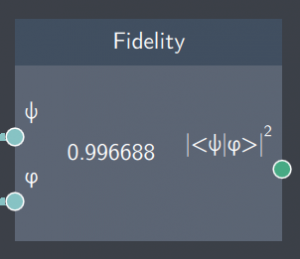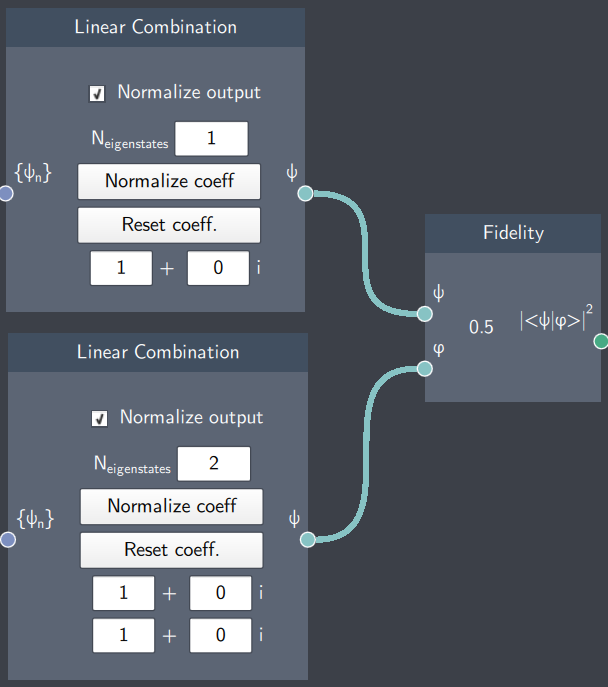# Fidelity

## Description

This node calculate the fidelity $F = |\langle \psi | \varphi \rangle |^2$ between two states. That is, the node calculates the norm-squared of the overlap of any two states $\psi$ and $\varphi$. This can be thought of as a measure of how much two states overlap.## Inputs

The node has the following inputs:

• $\psi$: State 1
• $\varphi$: State 2

## Content

The node displays the norm-square of the overlap between the states $\psi$ and $\varphi$ provided as inputs.

## Output

• Fidelity ($\langle \psi | \varphi \rangle |^2$): The fidelity of the states $\psi$ and $\varphi$ provided as inputs.

## Example

In the example below, the fidelity between a stationary state and a non-stationary (linear combination of the first two eigenstates) state is calculated using the Fidelity node.